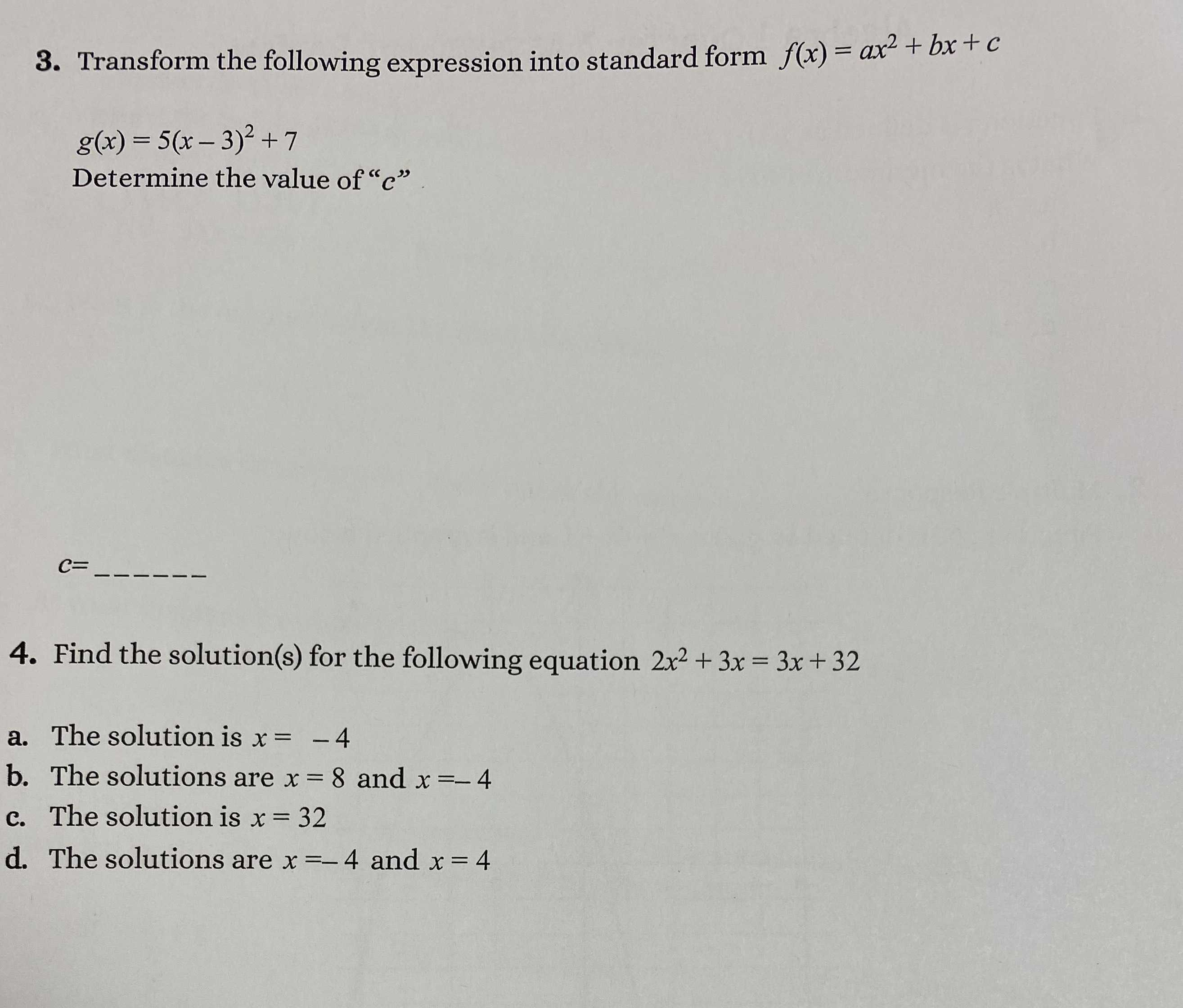### ¿Todavía tienes preguntas de matemáticas?

Pregunte a nuestros tutores expertos
Algebra
Pregunta3. Transform the following expression into standard form $$f ( x ) = a x ^ { 2 } + b x + c$$

$$g ( x ) = 5 ( x - 3 ) ^ { 2 } + 7$$

Determine the value of " $$c$$ "

$$5 ( x - 3 ) ^ { 2 } + 7 = 5 x ^ { 2 } - 30 x + 52$$
$$\therefore g ( x ) = 5 x ^ { 2 } - 30 x + 52$$
$$\Rightarrow c = 52$$